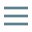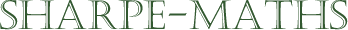Previous topic:
Equivalent fractions & cancelling
Current topic:
Mixed numbers & top heavy fractions
Next topic:

## Mixed numbers and top-heavy fractions

Up to this point, we have only looked at fractions between 0 and 1, which is a bit restricting. How do we deal with one and a half? Or two and three quarters?

There are two ways in which we can deal with fractions which are outside of the interval $[0, 1]$. The first is to use Mixed numbers and the other is to use top-heavy fractions. These are different forms of the same thing, so often we can use either of these notations, though they are both useful in the right context.

Top heavy fractions are sometimes called Improper fractions but this is becoming less common as the word improper has changed its meaning somewhat down the years.

### Mixed numbers

Mixed numbers are, as the name implies, a mixture of whole numbers and fractions. They are a mathematical form of the common English form, one and a half. But in mathematics, because we like to keep everything nice and brief, it comes out as $1\frac{1}{2}$. You should notice that the fraction is written after the whole number without using a sign of any sort. However, this is one situation where the sign which is missing, is a $+$ sign. When you see $1{\frac{1}{2}}$, you should think of it as $1 + {\frac{1}{2}}$. (Very much more often, if there is no sign between symbols, the missing sign is a $\times$, but we will talk about this more as we proceed.) This is one of the reasons why many mathematics teachers are not hugely fond of mixed numbers!

### Top heavy fractions

These are a little less obvious, but often much more useful. A top heavy fraction is a single fraction which combines the whole number part into the single fraction.

Top heavy fractions are those whose numerator is bigger than the denominator. A simple example might be $\frac{3}{2}$ which is three halves; two halves make one whole with one half left over, so we can write this as $1 \frac{1}{2}$.